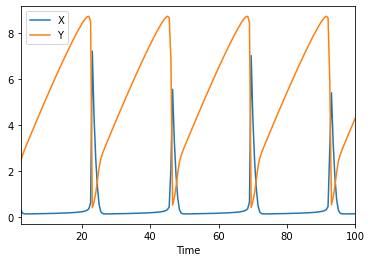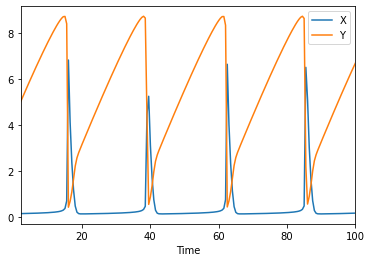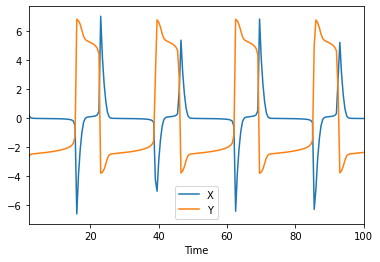# Simulating a model with basico

First some jupyter magic for plotting and convenience

:

%pylab
%matplotlib inline
import sys
if not '../..' in sys.path:
sys.path.append('../..')

Using matplotlib backend: Qt5Agg
Populating the interactive namespace from numpy and matplotlib


Now import basico

:

from basico import *


now we are ready to load a model, just adjust the file_name variable, to match yours. The file can be a COPASI or SBML file. For this example, we use the brusselator model, that is distributed with the package.

:

file_name = get_examples('brusselator')

:

model = load_model(file_name)


now we are ready to simulate. Calling run_time_course will run the simulation as specified in the COPASI file and return a pandas dataframe for it.

:

run_time_course().head()

:

X Y
Time
2.0 0.345934 2.368188
2.5 0.182762 2.658596
3.0 0.147433 2.863681
3.5 0.141129 3.048319
4.0 0.140734 3.228345

for plotting you would then just plot that as one does

:

df = run_time_course()
df.plot()

:

<AxesSubplot:xlabel='Time'>## The run_time_course command

you can change different options for the time course by adding named parameters into the run_time_course_command. Supported are:

• model: incase you want to use another model than the one last loaded

• scheduled: to mark the model as scheduled

• update_model: to update the initial state after the simulation is run

• duration: to specify how long the simulation is run

• automatic: in case you would like automatic step size being used

• output_event: in case you would like to have the event values before and after the event hit listed

• start_time: to change the start time

• step_number or intervals: to overwrite the number of steps being used

• method: a method name to use for the simulation.

so lets run two simulations that will be different slightly, as we will use the update_model flag:

:

df1 = run_time_course(update_model=True)
df2 = run_time_course(update_model=True)

:

df1.plot(), df2.plot()

:

(<AxesSubplot:xlabel='Time'>, <AxesSubplot:xlabel='Time'>)And now you could plot the difference between them too:

:

(df1-df2).plot()

:

<AxesSubplot:xlabel='Time'>:

(df1-df2).describe()

:

X Y
count 197.000000 197.000000
mean -0.014562 -0.123916
std 1.592729 3.615933
min -6.630084 -3.816620
25% -0.056936 -2.428037
50% -0.024780 -2.245888
75% 0.077394 4.837513
max 7.051794 6.844179
[ ]: